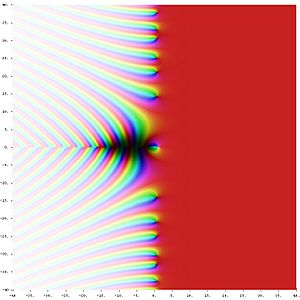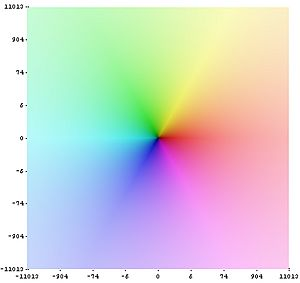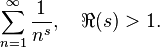# Riemann zeta function facts for kids

Kids Encyclopedia FactsRiemann zeta function ζ(s) in the complex plane. The color of a point s shows the value of ζ(s): strong colors are for values close to zero and hue encodes the value's argument. The white spot at s= 1 is the pole of the zeta function; the black spots on the negative real axis and on the critical line Re(s) = 1/2 are its zeros.The coloring of the complex function-values used above: positive real values are presented in red.

In mathematics, the Riemann zeta function, is a prominent function of great significance in number theory. It is named after German mathematician Bernhard Riemann. It is so important because of its relation to the distribution of prime numbers. It also has applications in other areas such as physics, probability theory, and applied statistics.

When using mathematical symbols to describe the Riemann zeta function, instead of explaining it using English, it is explained like this:$\sum_{n=1}^{\infty}\frac{1}{n^s}, \quad \Re(s)>1.$

s must be a complex number, meaning that it can involve the square root of -1, or i.

However, because this doesn't work for numbers where R(s) < 1, Riemann used some very clever mathematics known as analytic continuation, so that it would work for every number except 1 (where the function just gives you infinity).

Leonhard Euler discovered the first results about this function in the eighteenth century. It is named after Bernhard Riemann, who wrote about it in the memoir "On the Number of Primes Less Than a Given Quantity", published in 1859.

The Riemann hypothesis is a conjecture about the distribution of the zeros of the Riemann zeta function. Many mathematicians consider the Riemann hypothesis to be the most important unsolved problem in pure mathematics.

## Images for kidsRiemann zeta function Facts for Kids. Kiddle Encyclopedia.